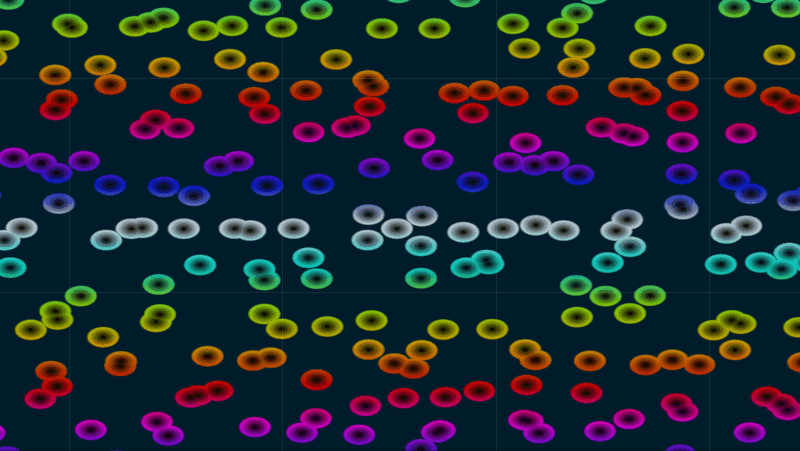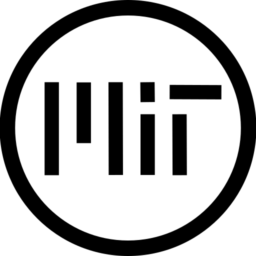# Floating Bubbles

Floating and colliding bubbles that can be colored with a gradient.

Story time: I’m working on a simple puzzle game and I’ve spent the last month or so finding shaders on this site and elsewhere: these shaders don’t have a big role in the game, they’re just cosmetics, so I didn’t want to spend time to learn how to write my own shaders. That said, I basically had to modify all of them to actually work and I already did customize a shader once with more uniforms and such, so I ended up still learning quite a lot about how shaders works.

At some point, though, there weren’t any more shaders I could use, so I had to actually try creating own of my own. I still didn’t want to learn more math, so I just tried starting with the worley noise snippet that this same site site provides. After a lot of trial and error, I ended up creating this shader, but I’m not sure how I did it. I’m sure someone more knowledgeable could be horrified by what I’ve created. End of story time.

The shader has some uniforms to easily customize the effect, but you can also try tinkering a bit with the rest of the code (read the comments in the code for more info!); for my case use, I used SCREEN_UV, but you’ll probably want to change to UV for most other cases.

You don’t need an actual gradient to color the bubbles, any image can go and you could also use one for the background with a little more effort. In the second and third screnshoots I used images where the colors change also vertically, instead of only horizontally like in a gradient, so the bubbles have their rims multicolored, resulting in a trippy effect.

``````/*
Floating Bubbles by PmkExpert
Create floating and colliding bubbles using worley and color them with a gradient

*/

uniform float speed = .5; //How fast the bubbles move
uniform sampler2D gradient; //The gradient texture used to color the bubbles; if it's repeated, it should start and end with the same color
uniform vec4 background_color : hint_color = vec4(0.0, 0.0, 0.0, 1.0);
uniform vec2 grid_vec = vec2(2.0, 2.0); //How to repeat the gradient
uniform vec2 grid_bubbles = vec2(20.0, 10.0); //How many bubbles; can also make them oval

vec2 random(vec2 uv) {
return vec2(fract(sin(dot(uv.xy,
vec2(12.9898,78.233))) * 43758.5453123));
}

float worley(vec2 uv, float columns, float rows) {

vec2 index_uv = floor(vec2(uv.x * columns, uv.y * rows));
vec2 fract_uv = fract(vec2(uv.x * columns, uv.y * rows));

float minimum_dist = 1.0;

for (int y= -1; y <= 1; y++) {
for (int x= -1; x <= 1; x++) {
vec2 neighbor = vec2(float(x),float(y));
vec2 point = random(index_uv + neighbor);
point = vec2( cos(TIME * point.x * speed), sin(TIME * point.y * speed) ) * 0.5 + 0.5;
vec2 diff = neighbor + point - fract_uv;
float dist = length(diff);
minimum_dist = min(minimum_dist, dist);
}
}

return minimum_dist;
}

vec2 grid(vec2 uv, float columns, float rows){
return fract(vec2(uv.x * columns, uv.y * rows));
}

void fragment() {
vec2 repeat = grid(SCREEN_UV, grid_vec.x, grid_vec.y); //Divide in grid, used to repeat the gradient
float worley = worley(SCREEN_UV, grid_bubbles.x, grid_bubbles.y); //Create bubbles
vec4 multi_color = texture(gradient, vec2(repeat.y, worley)); //Use gradient colors; you can change to repeat.x for a horizontal repeat
//You can change the float values here to modify the bubbles; don't ask for a mathematical explanation, I don't know
COLOR.rgb = mix(vec3(worley) * multi_color.rgb * 3.0, background_color.rgb , smoothstep(0.4, 0.5, worley * 1.5)); //The weight controls the bubbles' rim and size
COLOR.a = texture(TEXTURE, UV).a; //Preserve the original texture's transparency
}``````
###### Tags
2d, animated, bubble, canvas item, Color, worleyThe shader code and all code snippets in this post are under MIT license and can be used freely. Images and videos, and assets depicted in those, do not fall under this license. For more info, see our License terms.

## More from PmkExpert

### Hexagonal tiling + cog wheels